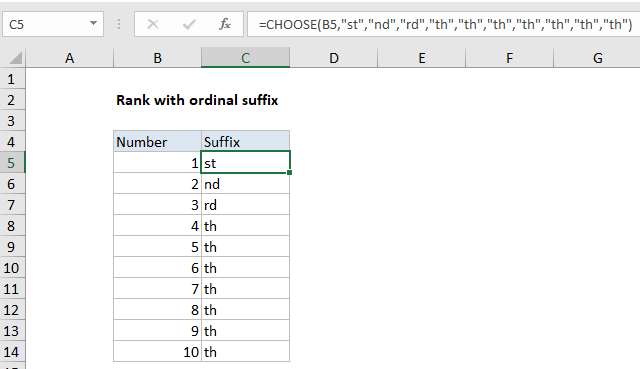## Excel Office

Excel How Tos, Tutorials, Tips & Tricks, Shortcuts

# Excel Rank with ordinal suffix Example

This tutorials shows how to Rank with ordinal suffix in Excel.

To add an ordinal suffix to a number (i.e. 1st, 2nd, 3rd, etc.) you can use a formula based on the CHOOSE function to assign the suffix.

## Formula

`=CHOOSE(number,"st","nd","rd","th","th","th","th","th","th","th")`## Explanation

In the example shown, the formula in C5 is:

`=CHOOSE(B5,"st","nd","rd","th","th","th","th","th","th","th")`

### How this formula works

Ordinal numbers represent position or rank in a sequential order. They are normally written using a number + letter suffix:  1st, 2nd, 3rd, etc.

Also See:   Custom weekday abbreviation in Excel

To get an ordinal suffix for a small set of numbers, you can use the CHOOSE function like this:

`=CHOOSE(B5,"st","nd","rd","th","th","th","th","th","th","th")`

Here CHOOSE simply picks up a number from column B and uses that number as an index to retrieve the right suffix.

### A universal formula

With a larger range of numbers it’s not practical to keep adding values to CHOOSE. In that case, you can switch to a more complicated formula that uses the MOD function:

```=IF(AND(MOD(ABS(A1),100)>10,MOD(ABS(A1),100)<14),"th",
CHOOSE(MOD(ABS(A1),10)+1,"th","st","nd","rd","th","th","th","th","th","th"))```

This formula first uses MOD with AND to “trap” the case of numbers like 11, 12, 13, 111, 112, 113, etc that have a non-standard suffix with is always “th”. All other numbers use the 10 suffix values inside CHOOSE.

Also See:   Get fiscal quarter from date in Excel

The ABS function is used to handle negative numbers as well as positive numbers.

### Concatenate suffix to number

You can concatenate (join) the suffix directly using either formula above. For example to add an ordinal suffix to a number 1-10 in A1:

`=A1&CHOOSE(A1,"st","nd","rd","th","th","th","th","th","th","th")`

But be aware that doing so will change the number into a text value.

Also See:   How to fill cell ranges with random number from fixed set of options in Excel